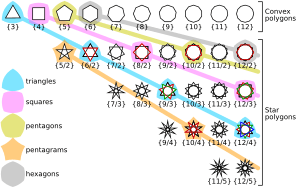# Regular polygon facts for kids

Kids Encyclopedia Facts

In Euclidean geometry, a regular polygon is a polygon that is equiangular (all angles are equal in measure) and equilateral (all sides have the same length). Regular polygons may be either convex or star. In the limit, a sequence of regular polygons with an increasing number of sides approximates a circle, if the perimeter or area is fixed, or a regular apeirogon (effectively a straight line), if the edge length is fixed.

## General propertiesRegular convex and star polygons with 3 to 12 vertices labelled with their Schläfli symbols

These properties apply to all regular polygons, whether convex or star.

A regular n-sided polygon has rotational symmetry of order n.

All vertices of a regular polygon lie on a common circle (the circumscribed circle); i.e., they are concyclic points. That is, a regular polygon is a cyclic polygon.

Together with the property of equal-length sides, this implies that every regular polygon also has an inscribed circle or incircle that is tangent to every side at the midpoint. Thus a regular polygon is a tangential polygon.

A regular n-sided polygon can be constructed with compass and straightedge if and only if the odd prime factors of n are distinct Fermat primes. See constructible polygon.de:Polygon#Regelmäßige PolygoneRegular polygon Facts for Kids. Kiddle Encyclopedia.